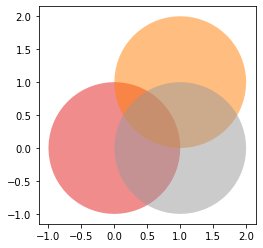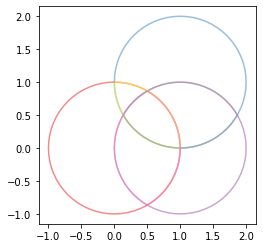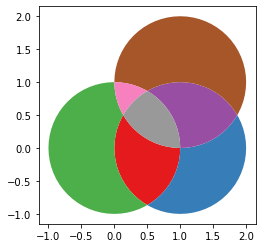# Overlay polygons ("cascaded intersection + difference")¶

Goal: given a set of overlapping polygons, create a new polygon layer with all polygons overlayed

Based on https://gis.stackexchange.com/a/326583/9828 (this is only all intersections, without the non-intersecting parts of the original polygons)

In :
%matplotlib inline

import geopandas
import shapely.geometry
import shapely.ops

import itertools

In :
circle1 = shapely.geometry.Point(0, 0).buffer(1)
circle2 = shapely.geometry.Point(1, 1).buffer(1)
circle3 = shapely.geometry.Point(1, 0).buffer(1)

In :
s = geopandas.GeoSeries([circle1, circle2, circle3])

In :
s.plot(alpha=.5, cmap='Set1')

Out:
<matplotlib.axes._subplots.AxesSubplot at 0x7fae967601d0>Calculate all pairwise intersections:

In :
all_intersections = [a.intersection(b) for a, b in list(itertools.combinations(s, 2))]


Combine those intersections with the original polygons:

In :
s_all = pd.concat([s, geopandas.GeoSeries(all_intersections)])


Then, we take the boundaries of all those polygons:

In :
s_all.boundary.plot(cmap='Set1', alpha=.5)

Out:
<matplotlib.axes._subplots.AxesSubplot at 0x7fae9467e7b8>From those boundaries, we can polygonize the union of all linestrings:

In :
polys = list(shapely.ops.polygonize(s_all.boundary.unary_union))

In :
polys = geopandas.GeoSeries(polys)

In :
polys.plot(cmap='Set1')

Out:
<matplotlib.axes._subplots.AxesSubplot at 0x7fae94605860>In [ ]: